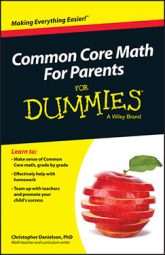##### Common Core Math For Parents For Dummies with Videos OnlineAlgebra can help to reveal how things are built — what different scenarios have in common with each other and what the important differences are. The Common Core State Standards refer to this idea as algebraic structure.

When you double the side lengths of a rectangle (or any geometric figure), but leave the angle measures the same, the area grows by a factor of 4. Triple the lengths and the area grows by a factor of 9. Doing so makes the relationship between length and area quadratic. Quadratic structure appears in many other guises — as the relationship between the number of vertices of a regular polygon and the number of its diagonals, for example, and as the result of multiplying two linear functions together. When you pay attention to the similarities of these various contexts, you notice that they have the same structure.

Noticing and using algebraic structure requires stepping back from your work and thinking about the big picture. In fact, doing this is a Standard for Mathematical Practice. Rather than looking at a chapter title in their textbook to decide what technique to use, students need to select techniques and ideas that make sense based on the relationships in the problem being considered.

Algebraic structure supports students working with mathematical modeling, which is an important part of the Common Core high school curriculum (and across all the grades). When students are building mathematical models, they're typically trying to understand something better that comes from outside the realm of mathematics. They're trying to answer a question that someone might reasonably ask outside of math class, and using mathematics to get a good answer. Part of building a good mathematical model involves seeing the structure of the situation. A student may ask questions such as "How are these variables related?" and "What other problems have been solved that involved similar relationships?" These questions draw the student back from the particulars of the context and help the student to focus on its mathematical structure.